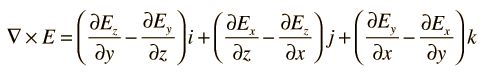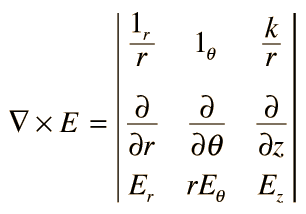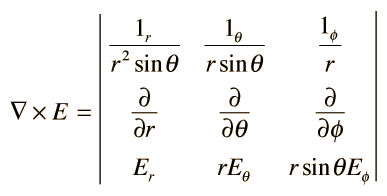# The Curl

The curl of a vector function is the vector product of the del operator with a vector function:where i,j,k are unit vectors in the x, y, z directions. It can also be expressed in determinant form:### Curl in cylindrical and sphericalcoordinate systems

 Applications: London equation for superconductors Maxwell's equations
Index

Vector calculus

 HyperPhysics*****HyperMath*****Calculus R Nave
Go Back

# Curl, Cylindrical

The curl in cylindrical polar coordinates, expressed in determinant form is:Index

Vector calculus

 HyperPhysics*****HyperMath*****Calculus R Nave
Go Back

# Curl, Spherical

The curl in spherical polar coordinates, expressed in determinant form is:Index

Vector calculus

 HyperPhysics*****HyperMath*****Calculus R Nave
Go Back